# Maharashtra Board Practice Set 10 Class 6 Maths Solutions Chapter 4 Operations on Fractions

Balbharti Maharashtra State Board Class 6 Maths Solutions covers the Std 6 Maths Chapter 4 Operations on Fractions Class 6 Practice Set 10 Answers Solutions.

## 6th Standard Maths Practice Set 10 Answers Chapter 4 Operations on Fractions

Question 1.
i. $$6 \frac{1}{3}+2 \frac{1}{3}$$
ii. $$1 \frac{1}{4}+3 \frac{1}{2}$$
iii. $$5 \frac{1}{5}+2 \frac{1}{7}$$
iv. $$3 \frac{1}{5}+2 \frac{1}{3}$$
Solution:
i. $$6 \frac{1}{3}+2 \frac{1}{3}$$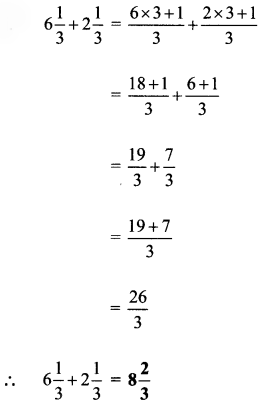ii. $$1 \frac{1}{4}+3 \frac{1}{2}$$iii. $$5 \frac{1}{5}+2 \frac{1}{7}$$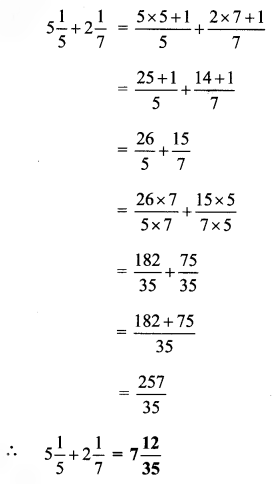iv. $$3 \frac{1}{5}+2 \frac{1}{3}$$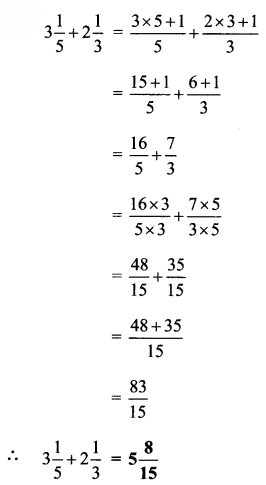Question 2.
Subtract:
i. $$3 \frac{1}{3}-1 \frac{1}{4}$$
ii. $$5 \frac{1}{2}-3 \frac{1}{3}$$
iii. $$7 \frac{1}{8}-6 \frac{1}{10}$$
iv. $$7 \frac{1}{2}-3 \frac{1}{5}$$
Solution:
i. $$3 \frac{1}{3}-1 \frac{1}{4}$$ii. $$5 \frac{1}{2}-3 \frac{1}{3}$$iii. $$7 \frac{1}{8}-6 \frac{1}{10}$$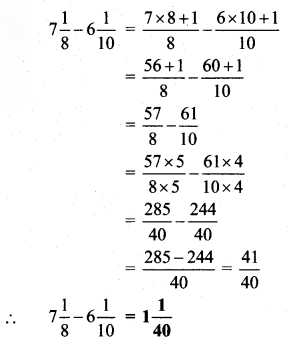iv. $$7 \frac{1}{2}-3 \frac{1}{5}$$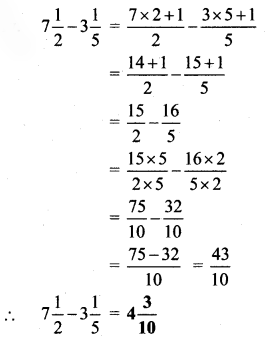Question 3.
Solve:
i. Suyash bought $$2\frac { 1 }{ 2 }$$ kg of sugar and Ashish bought $$3\frac { 1 }{ 2 }$$ kg. How much sugar did they buy altogether? If sugar costs Rs 32 per kg, how much did they spend on the sugar they bought?

ii. Aradhana grows potatoes in $$\frac { 2 }{ 5 }$$ part of her garden, greens in $$\frac { 1 }{ 3 }$$ part and brinjals in the remaining part. On how much of her plot did she plant brinjals?

iii. Sandeep filled water in $$\frac { 4 }{ 7 }$$ of an empty tank. After that, Ramakant filled $$\frac { 1 }{ 4 }$$ part more of the same tank. Then Umesh used $$\frac { 3 }{ 14 }$$ part of the tank to water the garden. If the tank has a maximum capacity of 560 litres, how many litres of water will be left in the tank?
Solution:
i. Sugar bought by Suyash = $$2\frac { 1 }{ 2 }$$ kg
Sugar bought by Ashish = $$3\frac { 1 }{ 2 }$$ kg
∴ Total sugar bought by both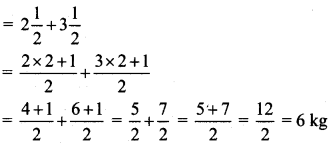Cost of 1 kg of sugar = Rs 32
∴ Cost of 6 kg of sugar = 32 x 6
= Rs 192
∴ They bought 6 kg sugar altogether and the total money spent on sugar is Rs 192.

ii. Part of garden occupied by potatoes = $$\frac { 2 }{ 5 }$$
Part of garden occupied by greens = $$\frac { 1 }{ 3 }$$
Since brinjals are planted in the remaining part,
∴ (Part occupied by potatoes) + (part occupied by greens) + (part occupied by brinjals) = 1 entire garden.
∴ Part of garden occupied by brinjals = 1 – (part of garden occupied by potatoes + part of garden occupied by greens)∴ Aradhana planted brinjals on $$\frac { 4 }{ 15 }$$ part of her plot.

iii. Part of tank filled by Sandeep = $$\frac { 4 }{ 7 }$$
Part of tank filled by Ramakant = $$\frac { 1 }{ 4 }$$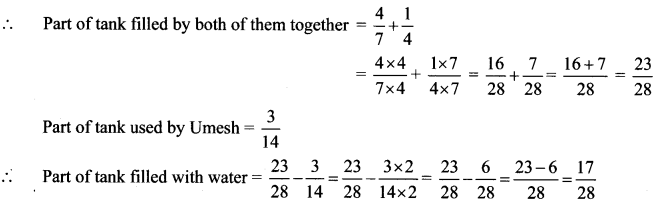Since maximum capacity of tank is 560 litres
∴ Quantity of water left in tank = $$\frac { 17 }{ 28 }\times560$$ = 340 litres
∴ The quantity of water left in the tank is 340 litres.

#### Maharashtra Board Class 6 Maths Chapter 4 Operations on Fractions Practice Set 10 Intext Questions and Activities

Question 1.
How to do this subtraction: $$4 \frac{1}{4}-2 \frac{1}{2}$$ ? Is it same as $$\left[4-2+\frac{1}{4}-\frac{1}{2}\right]$$ ? (Textbook pg. no. 23)
Solution:
$$4 \frac{1}{4}-2 \frac{1}{2}$$$$\left[4-2+\frac{1}{4}-\frac{1}{2}\right]$$The subtraction $$4 \frac{1}{4}-2 \frac{1}{2}$$ is the same as $$\left[4-2+\frac{1}{4}-\frac{1}{2}\right]$$.

Scroll to Top